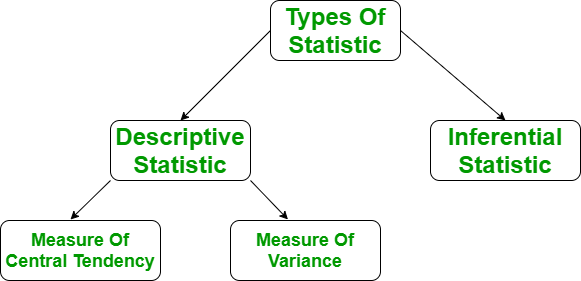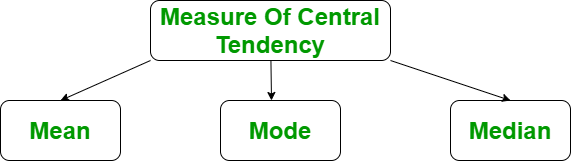Open in App
Not now

# Statistics with Python

• Difficulty Level : Hard
• Last Updated : 27 Sep, 2021

Statistics, in general, is the method of collection of data, tabulation, and interpretation of numerical data. It is an area of applied mathematics concern with data collection analysis, interpretation, and presentation. With statistics, we can see how data can be used to solve complex problems.

In this tutorial, we will learn about solving statistical problems with Python and will also learn the concept behind it. Let’s start by understanding some concepts that will be useful throughout the article.

Note: We will be covering descriptive statistics with the help of the statistics module provided by Python.

## Understanding the Descriptive Statistics

In a layman’s term, descriptive statistics generally means describing the data with the help of some representative methods like charts, tables, Excel files, etc. The data is described in such a way that it can express some meaningful information that can also be used to find some future trends. Describing and summarizing a single variable is called univariate analysis. Describing a statistical relationship between two variables is called bivariate analysis. Describing the statistical relationship between multiple variables is called multivariate analysis.

There are two types of descriptive Statistics –

• Measure of central tendency
• Measure of variability## Measure of central tendency

The measure of central tendency is a single value that attempts to describe the whole set of data. There are three main features of central tendency –

• Mean
• Median
• Median Low
• Median High
• Mode### Mean

It is the sum of observations divided by the total number of observations. It is also defined as average which is the sum divided by count.The mean() function returns the mean or average of the data passed in its arguments. If passed argument is empty, StatisticsError is raised.

Example:

## Python3

 # Python code to demonstrate the working of# mean() # importing statistics to handle statistical# operationsimport statistics # initializing listli = [1, 2, 3, 3, 2, 2, 2, 1] # using mean() to calculate average of list# elementsprint ("The average of list values is : ",end="")print (statistics.mean(li))

Output:

The average of list values is : 2

### Median

It is the middle value of the data set. It splits the data into two halves. If the number of elements in the data set is odd then the center element is median and if it is even then the median would be the average of two central elements.

For Odd Numbers:For Even Numbers:median() function is used to calculate the median, i.e middle element of data. If the passed argument is empty, StatisticsError is raised.

Example:

## Python3

 # Python code to demonstrate the# working of median() on various# range of data-sets # importing the statistics modulefrom statistics import median # Importing fractions module as frfrom fractions import Fraction as fr # tuple of positive integer numbersdata1 = (2, 3, 4, 5, 7, 9, 11) # tuple of floating point valuesdata2 = (2.4, 5.1, 6.7, 8.9) # tuple of fractional numbersdata3 = (fr(1, 2), fr(44, 12),        fr(10, 3), fr(2, 3)) # tuple of a set of negative integersdata4 = (-5, -1, -12, -19, -3) # tuple of set of positive# and negative integersdata5 = (-1, -2, -3, -4, 4, 3, 2, 1) # Printing the median of above datasetsprint("Median of data-set 1 is % s" % (median(data1)))print("Median of data-set 2 is % s" % (median(data2)))print("Median of data-set 3 is % s" % (median(data3)))print("Median of data-set 4 is % s" % (median(data4)))print("Median of data-set 5 is % s" % (median(data5)))

Output:

Median of data-set 1 is 5
Median of data-set 2 is 5.9
Median of data-set 3 is 2
Median of data-set 4 is -5
Median of data-set 5 is 0.0

### Median Low

median_low() function returns the median of data in case of odd number of elements, but in case of even number of elements, returns the lower of two middle elements. If the passed argument is empty, StatisticsError is raised

Example:

## Python3

 # Python code to demonstrate the# working of median_low() # importing the statistics moduleimport statistics # simple list of a set of integersset1 = [1, 3, 3, 4, 5, 7] # Print median of the data-set # Median value may or may not# lie within the data-setprint("Median of the set is % s"    % (statistics.median(set1))) # Print low median of the data-setprint("Low Median of the set is % s "    % (statistics.median_low(set1)))

Output:

Median of the set is 3.5
Low Median of the set is 3 

### Median High

median_high() function returns the median of data in case of odd number of elements, but in case of even number of elements, returns the higher of two middle elements. If passed argument is empty, StatisticsError is raised.

Example:

## Python3

 # Working of median_high() and median() to# demonstrate the difference between them. # importing the statistics moduleimport statistics # simple list of a set of integersset1 = [1, 3, 3, 4, 5, 7] # Print median of the data-set # Median value may or may not# lie within the data-setprint("Median of the set is %s"    % (statistics.median(set1))) # Print high median of the data-setprint("High Median of the set is %s "    % (statistics.median_high(set1)))

Output:

Median of the set is 3.5
High Median of the set is 4 

### Mode

It is the value that has the highest frequency in the given data set. The data set may have no mode if the frequency of all data points is the same. Also, we can have more than one mode if we encounter two or more data points having the same frequency.

mode() function returns the number with the maximum number of occurrences. If the passed argument is empty, StatisticsError is raised.

Example:

## Python3

 # Python code to demonstrate the# working of mode() function# on a various range of data types # Importing the statistics modulefrom statistics import mode # Importing fractions module as fr# Enables to calculate harmonic_mean of a# set in Fractionfrom fractions import Fraction as fr # tuple of positive integer numbersdata1 = (2, 3, 3, 4, 5, 5, 5, 5, 6, 6, 6, 7) # tuple of a set of floating point valuesdata2 = (2.4, 1.3, 1.3, 1.3, 2.4, 4.6) # tuple of a set of fractional numbersdata3 = (fr(1, 2), fr(1, 2), fr(10, 3), fr(2, 3)) # tuple of a set of negative integersdata4 = (-1, -2, -2, -2, -7, -7, -9) # tuple of stringsdata5 = ("red", "blue", "black", "blue", "black", "black", "brown")  # Printing out the mode of the above data-setsprint("Mode of data set 1 is % s" % (mode(data1)))print("Mode of data set 2 is % s" % (mode(data2)))print("Mode of data set 3 is % s" % (mode(data3)))print("Mode of data set 4 is % s" % (mode(data4)))print("Mode of data set 5 is % s" % (mode(data5)))

Output:

Mode of data set 1 is 5
Mode of data set 2 is 1.3
Mode of data set 3 is 1/2
Mode of data set 4 is -2
Mode of data set 5 is black

Refer to the below article to get detailed information about averages and Measures of central tendency.

## Measure of variability

Till now, we have studied the measure of central tendency but this alone is not sufficient to describe the data. To overcome this we need the measure of variability. Measure of variability is known as the spread of data or how well is our data is distributed. The most common variability measures are:

• Range
• Variance
• Standard deviation### Range

The difference between the largest and smallest data point in our data set is known as the range. The range is directly proportional to the spread of data that means the bigger the range, more the spread of data and vice versa.

Range = Largest data value – smallest data value

We can calculate the maximum and minimum value using the max() and min() methods respectively.

Example:

## Python3

 # Sample Dataarr = [1, 2, 3, 4, 5] #Finding MaxMaximum = max(arr)# Finding MinMinimum = min(arr) # Difference Of Max and MinRange = Maximum-Minimum    print("Maximum = {}, Minimum = {} and Range = {}".format(    Maximum, Minimum, Range))

Output:

Maximum = 5, Minimum = 1 and Range = 4

### Variance

It is defined as an average squared deviation from the mean. It is being calculated by finding the difference between every data point and the average which is also known as the mean, squaring them, adding all of them, and then dividing by the number of data points present in our data set.where N = number of terms

u = Mean

The statistics module provides the variance() method that does all the maths behind the scene. If passed argument is empty, StatisticsError is raised.

Example:

## Python3

 # Python code to demonstrate variance()# function on varying range of data-types # importing statistics modulefrom statistics import variance # importing fractions as parameter valuesfrom fractions import Fraction as fr # tuple of a set of positive integers# numbers are spread apart but not very muchsample1 = (1, 2, 5, 4, 8, 9, 12) # tuple of a set of negative integerssample2 = (-2, -4, -3, -1, -5, -6) # tuple of a set of positive and negative numbers# data-points are spread apart considerablysample3 = (-9, -1, -0, 2, 1, 3, 4, 19) # tuple of a set of fractional numberssample4 = (fr(1, 2), fr(2, 3), fr(3, 4),           fr(5, 6), fr(7, 8)) # tuple of a set of floating point valuessample5 = (1.23, 1.45, 2.1, 2.2, 1.9) # Print the variance of each samplesprint("Variance of Sample1 is % s " % (variance(sample1)))print("Variance of Sample2 is % s " % (variance(sample2)))print("Variance of Sample3 is % s " % (variance(sample3)))print("Variance of Sample4 is % s " % (variance(sample4)))print("Variance of Sample5 is % s " % (variance(sample5)))

Output:

Variance of Sample1 is 15.80952380952381
Variance of Sample2 is 3.5
Variance of Sample3 is 61.125
Variance of Sample4 is 1/45
Variance of Sample5 is 0.17613000000000006 

### Standard Deviation

It is defined as the square root of the variance. It is being calculated by finding the Mean, then subtract each number from the Mean which is also known as average and square the result. Adding all the values and then divide by the no of terms followed the square root.where N = number of terms

u = Mean

stdev() method of the statistics module returns the standard deviation of the data. If passed argument is empty, StatisticsError is raised.

Example:

## Python3

 # Python code to demonstrate stdev()# function on various range of datasets # importing the statistics modulefrom statistics import stdev # importing fractions as parameter valuesfrom fractions import Fraction as fr # creating a varying range of sample sets# numbers are spread apart but not very muchsample1 = (1, 2, 5, 4, 8, 9, 12) # tuple of a set of negative integerssample2 = (-2, -4, -3, -1, -5, -6) # tuple of a set of positive and negative numbers# data-points are spread apart considerablysample3 = (-9, -1, -0, 2, 1, 3, 4, 19) # tuple of a set of floating point valuessample4 = (1.23, 1.45, 2.1, 2.2, 1.9) # Print the standard deviation of# following sample sets of observationsprint("The Standard Deviation of Sample1 is % s"      % (stdev(sample1))) print("The Standard Deviation of Sample2 is % s"      % (stdev(sample2))) print("The Standard Deviation of Sample3 is % s"      % (stdev(sample3)))  print("The Standard Deviation of Sample4 is % s"      % (stdev(sample4)))

Output:

The Standard Deviation of Sample1 is 3.9761191895520196
The Standard Deviation of Sample2 is 1.8708286933869707
The Standard Deviation of Sample3 is 7.8182478855559445
The Standard Deviation of Sample4 is 0.41967844833872525

Refer to the below article to get detailed information about the Measure of variability.

My Personal Notes arrow_drop_up